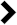# Akai GX 600 D Service Manual

This is the 74 pages manual for Akai GX 600 D Service Manual.

Page: 1 / 74## Extracted text from Akai GX 600 D Service Manual (Ocr-read)

### Page 25

VII. OPERATING PRINCIPALS OF DOLBY NR CIRCUIT

G)
T'Rl TR2 -�-P-F'
INPUT 9 -
em (a)
| I]
e: 9
ea
m3
TR4 (a)
OUTPUTO-�_-
eout k0
_eI+e2 OFF ON

DOLBY NR. SWITCH

INPUT SIGNAL LEVEL
ein

SIGNAL LEVEL AT POINT (b)
6| : ; q ;

SIGNAL LEVEL AT POINT (c)
9:

ourpu-�r SIGNAL LEVEL
eom - e. + e,

Fig. 32 Level and Phase of
each point at individual frequency.

_1. RECORDING DOLBY NR (Figs. 31, 32)

In figure 31, the Input Signal e in passes TRl ~ TR4
and becomes the output signal. The signal from TRZ
passes the High Pass Filter (H.P.F.) and is ampliﬁed
at TR6, TR7. At this time, TR6 signal is controlled
by FET (field effect transistor) TR5 gate DC. bias
and the signal from TR7 is varied by means of
Diodes D3, D4 characteristics and is emitted as e;.
This output signal e; is inphased with TRZ output
signal and TR4 output becomes the dolbyized output
- signal e out.

Accordingly if input signal level e in is small,
because FET TR5 becomes an electronic attenuator
with a certain impedance value, the output signal
02 from (C) point is attenuated by H.P.F. and TR5
and becomes e/k. Then.it is amplified at TR6, TR7.
With A representing this ampliﬁcation, e2 becomes
as shown in formula (1) below.

e2=e/k-A-l ...... (1)

Fig. 31 Recording Dolby NR diagram

Where 1 is the determined attenuated volume by
means of Diode D3, D4 characteristics, if

A.K - 1 = m, formula (1) becomes as
shown in formula (2) below:

e; = me ....... (2)

Because e; of formula (2) and TR2 output signal
er is composited, this output signal e out becomes
as showu in formula (3) below:

eout=e1+e2=e1+me ....... (3)

In formula (3) above, the relation of e, and me with
regards to the Dolby NR System, is the relation
between e out and e1, i.e., the value of e out is
10 dB higher than e1. For this reason, at a level of
-30 dB lower than the Dolby level, the output
signal is 10 dB higher than the more than 400 Hz
input signal e in.

Also, if the input signal e in level is high, it is
ampliﬁed at TR6, current modified at diode D5, and
the D.C. Voltage at (d) point is also large in proportion
to input.

Accordingly, if e3 becomes large, FET TR5 im-
pedance is reduced and (c) point signal level e2
gradually becomes smaller. Thus at a level higher
than the Dolby level, formula (4) below is applicable:

Eout=e1+me=e ...... (4)
e > me
Consequently, the input and output levels display
linear characteristics.

NOTE: H.P.F. (high pass ﬁlter)
This filter attenuates an under 400 Hz
signal at 18 dB octave.

24

### Page 42

FIG. 3

ILLUSTRATION OF REEL MOTOR/REEL TABLE BLOCK

WM/

41